# Spencer cohomology

The de Rham cohomology and Dolbeault cohomology (cf. Duality in complex analysis) can be viewed as cohomologies with coefficients in the sheaf of locally constant, respectively harmonic, functions. Spencer cohomology is a generalization of these two cohomologies for the case of the solution sheaf of an arbitrary linear differential operator.

Namely, letand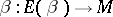be smooth vector bundles (cf. also Vector bundle) and letbe a linear differential operator acting from the module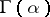of smooth sections ofto the module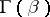. Denote by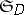the sheaf of solutions of. To find the cohomology ofwith coefficients inone needs a resolvent of the sheaf.

Spencer cohomology appears as a result of constructing a resolvent by a locally exact complex of differential operators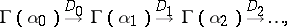where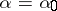,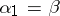,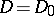. The condition that the complex be locally exact is too strong, and therefore D. Spencer proposed the weaker condition that the complex should be "formally exact" . In this setting, there exists for a formally integrable differential operatora canonical construction ([a5], [a6], [a1]) of a complex, called the second (or sophisticated) Spencer complex. In this complex,the vector bundles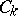have the form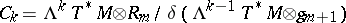, whereare prolongations of the differential equation corresponding to(cf. also Prolongation of solutions of differential equations) andare the symbols of these prolongations (cf. also Symbol of an operator). The differential operatorsare first-order partial differential operators whose symbols are induced by the exterior multiplication.

The-Poincaré lemma [a6] shows that the cohomology of the complex does not depend onwhenis large enough. The stable cohomology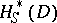is called the Spencer cohomology of the differential operator.

In general, the second Spencer complex does not produce a resolvent of; however, it does in certain special cases, e.g. whenis analytical operator [a6].

Almost-all cohomologies encountered in applications are of Spencer type. For example, de Rham cohomology corresponds to the differential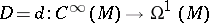, and the Dolbeault cohomology corresponds to the Cauchy–Riemann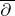-operator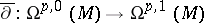. Ifis a determined operator such that not all covectors are characteristic, then,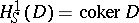and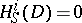for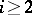. In general,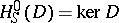for each formally integrable operators.

In the case of Lie equations and corresponding geometrical structures (see [a2]), the first Spencer cohomology gives an estimate of the set of deformations of the structure.

Ifis an elliptic partial differential operator (cf. Elliptic partial differential equation) andis a compact manifold, then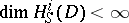and the Euler characteristic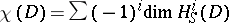of the Spencer complex is called the index of(cf. also Index formulas; Index theory). For elliptic Lie equations the index can be expressed in terms of characteristic classes corresponding to the geometrical structure ([a3]).

As is well-known, there are two main methods for calculating the de Rham cohomology: the Leray–Serre spectral sequence (cf. also Spectral sequence) and the theorem on coincidence of de Rham cohomology with invariant cohomology on homogeneous manifolds. These methods also apply to Spencer cohomology, provided the operatorsatisfies certain extra conditions.

Thus, if the base manifoldis the total space of a smooth bundle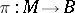over a simply-connected manifoldand if the fibres ofare not characteristic for, then there exists a spectral sequence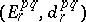converging to the Spencer cohomology; its second term is, where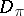is the fibrewise differential operator corresponding to[a4].

If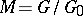is a homogeneous manifold and the structure groupis a compact connected Lie group of symmetries of, then [a4] the Spencer cohomologycoincides with the cohomology of the-invariant Spencer complex if the non-trivial characters of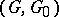are non-characteristic.